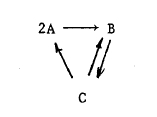# Contents

## Overview

A Petri Net is a kind of graph which can be used to simulate discrete processes. There are two kinds of nodes: (1) places, aka states, and (2) transitions. First we will explain the concept using the “place” metaphor. Think of each place as a tray that can hold a finite number of “tokens.” Each transition node is connected to a set of input place nodes, and a set of output place nodes. When the transition “fires,” it removes one token from each of its input places, and adds one token to each of its output places. So it is a kind of dataflow network, where there is just one datatype, the token.

Note that one transition may take multiple inputs from the same place, and have multiple outputs to the same place. For example, suppose transition t takes two inputs from p, and sends to outputs to q. Then when it fires, it will remove 2 tokens from p, and add two tokens to q.

The state of the network as a whole is given by a mapping from the set of place nodes to the natural numbers N. This is called a marking.

The state metaphor comes from applications such as chemical reaction networks. Picture a fixed collection of atoms, that are assembled into various types of molecules. Each state refers to a type of molecule. The number of tokens associated with a state (place) indicates how many of that type of molecule are in the system. So, taking the H2O example from the main Petri Net page, we have states for H, O and H2O, and a single transition with inputs at H and O, and an output at H2O. The firing of the transition corresponds to the formation of one H2O molecule.

Note that the transition structure of the net is non-deterministic. In any given configuration, there will be a subset of the transitions that are enabled to fire: namely, all those transitions for which every input place contains at least one token. So there are multiple ways to order the firings of the transition rules.

The representation can be enriched with probabilistic parameters to control the firing of the transitions. Then you could imagine the problem of finding equilibrirum states of the net.

$f_2 : x_2 \to 1$

Here $1$ denotes the tensor product of no objects.

tensor product symbol is written ‘$+$’ rather than ‘$\otimes$’.

For more on this, see:

## Stochastic Petri net

If we use $x_1$ to denote “rabbit” and $x_2$ to denote “fox”, the transitions are:

$birth : x_1 \to x_1 \otimes x_1$

in which one rabbit becomes two,

$predation : x_1 \otimes x_2 \to x_2 \otimes x_2$

in which a fox eats a rabbit and reproduces, resulting in two foxes, and

$death : x_2 \to 1$

in which one fox dies. Of course this model is ridiculous — it would be slightly less silly if we replaced ‘fox’ and ‘rabbit’ by asexual micro-organisms, one

• $\alpha$ is the birth rate,

Let $[x_1]$ denote the concentration of rabbits (say, in rabbits per hectare) and let $[x_2]$ the concentration of

(1)$\frac{d [x_1]}{ d t} = \alpha [x_1] - \beta [x_1] [x_2]$
(2)$\frac{d [x_2]}{ d t} = + \beta [x_1] [x_2] - \gamma [x_2]$

The standard procedure works as follows:

• Each transition contributes to the time derivative of $[x_i]$ in the obvious way: we take the rate at which that transition occurs, and multiply it by the number of times $x_i$ occurs as an output of that transition, minus the number of copies times it occurs as an input.

Chemical reaction networks are diagrams widely used in chemistry. They are quite similar to Petri nets (see below), but in some sense more primitive and less expressive:below, $A, B$ and $C$ are three molecular species, and we have four reactions between them:

$A + A \to B$
$C \to A + A$
$B \to C$
$C \to B$

and then try:

The last is apparently not free online, but here’s a quote that explains the philosophy:

The formal kineticist, on the other hand, takes a macroscopic viewpoint and his primitive concept is the elementary reaction. This is defined by a set of stoichiometric coefficients, together with a rule relating The abstract is also useful:

Abstract: The familiar idea of mass action kinetics is extended to embrace situations more general than chemically reacting mixtures in closed vessels. Thus, for

Abstract: Abstract bifurcation theory is one of the most widely used approaches for analysis of dynamical

• B.L. Clarke and I. Prigogine, Stability of complex reaction networks, Adv. Chem. Phys., Wiley, New York, 43 (1980), 1–-216.

There is an interesting relation between chemical reaction networks (or, better, Petri nets) and toric varieties. See:

Besides the works listed above, some good general starting-points include:

For a detailed introduction to stochastic Petri nets, see:

code/stub gen (if i remember correctly. These are also other types of Open source packages on wikipedia: